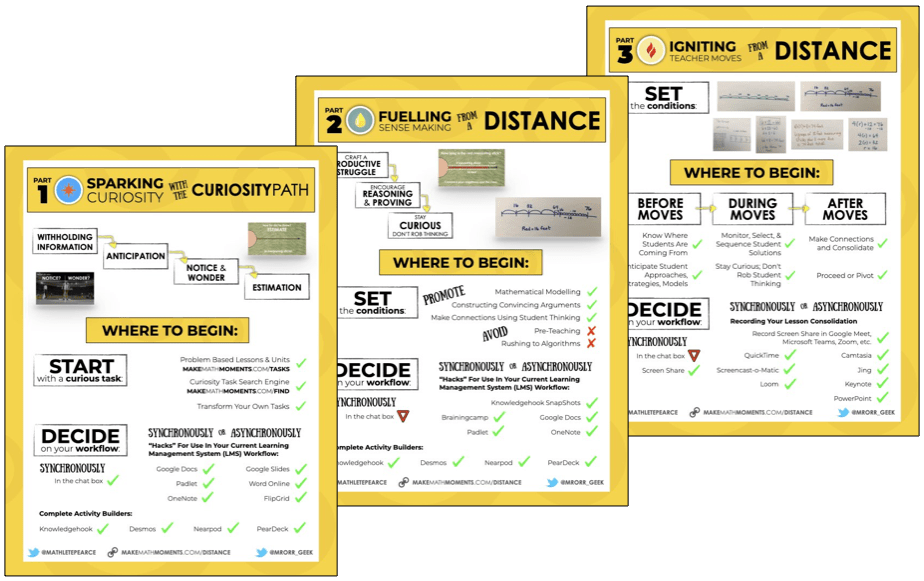# Category - Algebra

## Solving Two-Step Equations Visually Part 2

In this series of Math Is Visual Prompts, we slowly introduce the idea of Solving Two-Step Equations Using Partitive and Quotative Division Scenarios.

## Solving Two-Step Equations Visually

In this series of Math Is Visual Prompts, we slowly introduce the idea of Solving Two-Step Equations Using Partitive Division Scenarios.

## Solving One-Step Equations Visually

In this series of Math Is Visual Prompts, we slowly introduce the idea of Solving One-Step Equations Using Partitive and Quotative Division.